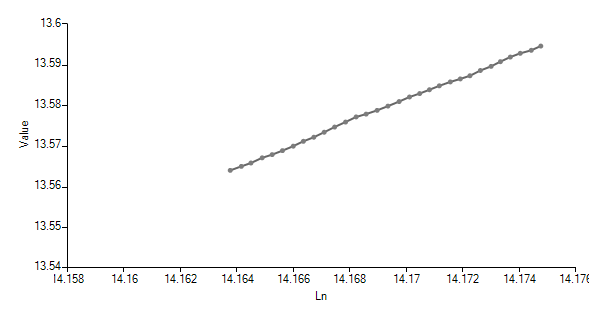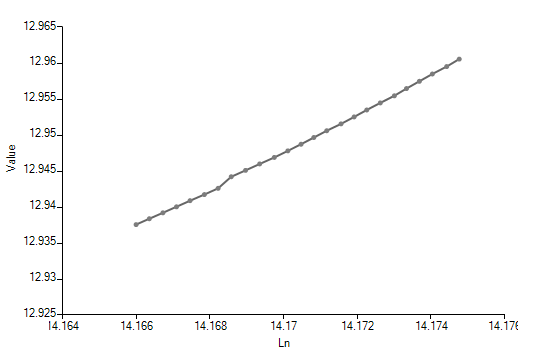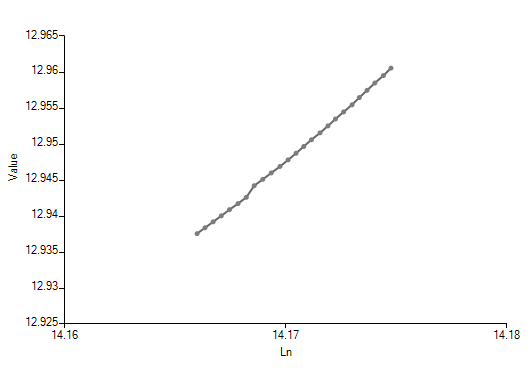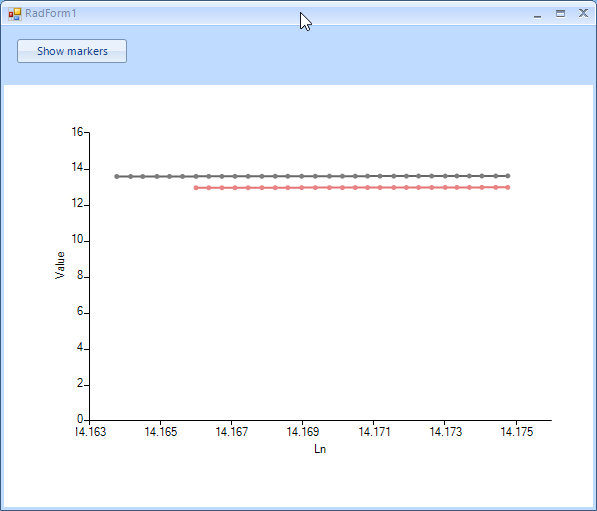# Horizontal LinearAxis

5 posts, 0 answers
1. ##### Valery65 posts
Member since:
May 2011

Posted 28 Apr 2020 Link to this post

I need the horizontal axis to be linear in the chart. For example:

public partial class Form1 : Form
{
public Form1()
{
InitializeComponent();
MakeChart();
}

private DataTable _dtChart;

private void MakeChart()
{
_dtChart = new DataTable("Chart");
var column = new DataColumn("COLUMN0", typeof(DateTime));
_dtChart.Columns.Add(column);
column = new DataColumn("COLUMN1", typeof(double));
_dtChart.Columns.Add(column);
column = new DataColumn("COLUMN2", typeof(double));
_dtChart.Columns.Add(column);
column = new DataColumn("COLUMN3", typeof(double));
_dtChart.Columns.Add(column);
_dtChart.BeginLoadData();
var row = new object[_dtChart.Columns.Count];
var date = new DateTime(2007, 1, 1);
row = date; row = 14.163764; row = 13.56391907;
_dtChart.Rows.Add(row);
row = date.AddDays(1); row = 14.16415691; row = 13.56490612;
_dtChart.Rows.Add(row);
row = date.AddDays(2); row = 14.16449642; row = 13.56572723;
_dtChart.Rows.Add(row);
row = date.AddDays(3); row = 14.16489506; row = 13.56701374;
_dtChart.Rows.Add(row);
row = date.AddDays(4); row = 14.16524887; row = 13.56779575;
_dtChart.Rows.Add(row);
row = date.AddDays(5); row = 14.16561508; row = 13.56877995;
_dtChart.Rows.Add(row);
row = date.AddDays(6); row = 14.1659956;  row = 13.56987286; row = 12.9375058;
_dtChart.Rows.Add(row);
row = date.AddDays(7); row = 14.16635513; row = 13.57107353; row = 12.93832116;
_dtChart.Rows.Add(row);
row = date.AddDays(8); row = 14.16672421; row = 13.57207203; row = 12.93914642;
_dtChart.Rows.Add(row);
row = date.AddDays(9); row = 14.16709232; row = 13.57329559; row = 12.93998154;
_dtChart.Rows.Add(row);
row = date.AddDays(10); row = 14.1674614;  row = 13.57457256; row = 12.94082651;
_dtChart.Rows.Add(row);
row = date.AddDays(11); row = 14.16784954; row = 13.57580566; row = 12.94168131;
_dtChart.Rows.Add(row);
row = date.AddDays(12); row = 14.16822338; row = 13.57705879; row = 12.94254593;
_dtChart.Rows.Add(row);
row = date.AddDays(13); row = 14.16857815; row = 13.57775879; row = 12.9441519;
_dtChart.Rows.Add(row);
row = date.AddDays(14); row = 14.16896725; row = 13.57868004; row = 12.94503586;
_dtChart.Rows.Add(row);
row = date.AddDays(15); row = 14.16935158; row = 13.5797205;  row = 12.94592957;
_dtChart.Rows.Add(row);
row = date.AddDays(16); row = 14.16974831; row = 13.58084583; row = 12.94683301;
_dtChart.Rows.Add(row);
row = date.AddDays(17); row = 14.17011547; row = 13.58196545; row = 12.94774618;
_dtChart.Rows.Add(row);
row = date.AddDays(18); row = 14.17047501; row = 13.58280563; row = 12.94866904;
_dtChart.Rows.Add(row);
row = date.AddDays(19); row = 14.17082119; row = 13.58374691; row = 12.94960158;
_dtChart.Rows.Add(row);
row = date.AddDays(20); row = 14.17117214; row = 13.58471298; row = 12.95054378;
_dtChart.Rows.Add(row);
row = date.AddDays(21); row = 14.17156029; row = 13.5856638;  row = 12.95149562;
_dtChart.Rows.Add(row);
row = date.AddDays(22); row = 14.17191505; row = 13.58641052; row = 12.95245707;
_dtChart.Rows.Add(row);
row = date.AddDays(23); row = 14.17225742; row = 13.58718204; row = 12.95342813;
_dtChart.Rows.Add(row);
row = date.AddDays(24); row = 14.1726265;  row = 13.58848;    row = 12.95440876;
_dtChart.Rows.Add(row);
row = date.AddDays(25); row = 14.17300701; row = 13.5894928;  row = 12.95539896;
_dtChart.Rows.Add(row);
row = date.AddDays(26); row = 14.17333603; row = 13.59065437; row = 12.95639868;
_dtChart.Rows.Add(row);
row = date.AddDays(27); row = 14.17368698; row = 13.5917902;  row = 12.95740793;
_dtChart.Rows.Add(row);
row = date.AddDays(28); row = 14.17403889; row = 13.59270477; row = 12.95842667;
_dtChart.Rows.Add(row);
row = date.AddDays(29); row = 14.17442799; row = 13.59344292; row = 12.95945488;
_dtChart.Rows.Add(row);
row = date.AddDays(30); row = 14.17476845; row = 13.59449482; row = 12.96049254;
_dtChart.Rows.Add(row);
_dtChart.EndLoadData();
var horizontalAxis = new LinearAxis {Title = "Ln"};
var verticalAxis = new LinearAxis
{
AxisType = AxisType.Second,
Title = "Value"
};
var lineSeria = new LineSeries
{
DisplayMember = "COLUMN1",
ValueMember = "COLUMN2",
VerticalAxis = verticalAxis,
HorizontalAxis = horizontalAxis,
};
radChartView1.Series.Add(lineSeria);
radChartView1.DataSource = _dtChart;
radChartView1.Refresh();

}

}

But nothing is drawn on the chart, and the range of the horizontal axis is wrong.

What am I doing wrong?

2. ##### Nadya
Admin509 posts

Posted 29 Apr 2020 Link to this post

Hello Valery,

The provided code snippet is greatly appreciated.

Note that LineSeries plot their Categorical data points on Cartesian Area using one categorical and one numerical axis. If you have numeric values on both vertical and horizontal axes, it would be suitable to use a ScatterLineSeries. Each point from the line in the chart in ScatterLineSeries is defined by two numeric values – XValue and YValue for the horizontal and for the vertical axis respectively.

I modified the provided code in order to use ScatterLineSeries and the data is now displayed correctly:

```public RadForm2()
{
InitializeComponent();

MakeChart();
}

private DataTable _dtChart;
private void MakeChart()
{
_dtChart = new DataTable("Chart");
var column = new DataColumn("COLUMN0", typeof(DateTime));
_dtChart.Columns.Add(column);
column = new DataColumn("COLUMN1", typeof(double));
_dtChart.Columns.Add(column);
column = new DataColumn("COLUMN2", typeof(double));
_dtChart.Columns.Add(column);
column = new DataColumn("COLUMN3", typeof(double));
_dtChart.Columns.Add(column);
_dtChart.BeginLoadData();
var row = new object[_dtChart.Columns.Count];
var date = new DateTime(2007, 1, 1);
row = date; row = 14.163764; row = 13.56391907;
_dtChart.Rows.Add(row);
row = date.AddDays(1); row = 14.16415691; row = 13.56490612;
_dtChart.Rows.Add(row);
row = date.AddDays(2); row = 14.16449642; row = 13.56572723;
_dtChart.Rows.Add(row);
row = date.AddDays(3); row = 14.16489506; row = 13.56701374;
_dtChart.Rows.Add(row);
row = date.AddDays(4); row = 14.16524887; row = 13.56779575;
_dtChart.Rows.Add(row);
row = date.AddDays(5); row = 14.16561508; row = 13.56877995;
_dtChart.Rows.Add(row);
row = date.AddDays(6); row = 14.1659956; row = 13.56987286; row = 12.9375058;
_dtChart.Rows.Add(row);
row = date.AddDays(7); row = 14.16635513; row = 13.57107353; row = 12.93832116;
_dtChart.Rows.Add(row);
row = date.AddDays(8); row = 14.16672421; row = 13.57207203; row = 12.93914642;
_dtChart.Rows.Add(row);
row = date.AddDays(9); row = 14.16709232; row = 13.57329559; row = 12.93998154;
_dtChart.Rows.Add(row);
row = date.AddDays(10); row = 14.1674614; row = 13.57457256; row = 12.94082651;
_dtChart.Rows.Add(row);
row = date.AddDays(11); row = 14.16784954; row = 13.57580566; row = 12.94168131;
_dtChart.Rows.Add(row);
row = date.AddDays(12); row = 14.16822338; row = 13.57705879; row = 12.94254593;
_dtChart.Rows.Add(row);
row = date.AddDays(13); row = 14.16857815; row = 13.57775879; row = 12.9441519;
_dtChart.Rows.Add(row);
row = date.AddDays(14); row = 14.16896725; row = 13.57868004; row = 12.94503586;
_dtChart.Rows.Add(row);
row = date.AddDays(15); row = 14.16935158; row = 13.5797205; row = 12.94592957;
_dtChart.Rows.Add(row);
row = date.AddDays(16); row = 14.16974831; row = 13.58084583; row = 12.94683301;
_dtChart.Rows.Add(row);
row = date.AddDays(17); row = 14.17011547; row = 13.58196545; row = 12.94774618;
_dtChart.Rows.Add(row);
row = date.AddDays(18); row = 14.17047501; row = 13.58280563; row = 12.94866904;
_dtChart.Rows.Add(row);
row = date.AddDays(19); row = 14.17082119; row = 13.58374691; row = 12.94960158;
_dtChart.Rows.Add(row);
row = date.AddDays(20); row = 14.17117214; row = 13.58471298; row = 12.95054378;
_dtChart.Rows.Add(row);
row = date.AddDays(21); row = 14.17156029; row = 13.5856638; row = 12.95149562;
_dtChart.Rows.Add(row);
row = date.AddDays(22); row = 14.17191505; row = 13.58641052; row = 12.95245707;
_dtChart.Rows.Add(row);
row = date.AddDays(23); row = 14.17225742; row = 13.58718204; row = 12.95342813;
_dtChart.Rows.Add(row);
row = date.AddDays(24); row = 14.1726265; row = 13.58848; row = 12.95440876;
_dtChart.Rows.Add(row);
row = date.AddDays(25); row = 14.17300701; row = 13.5894928; row = 12.95539896;
_dtChart.Rows.Add(row);
row = date.AddDays(26); row = 14.17333603; row = 13.59065437; row = 12.95639868;
_dtChart.Rows.Add(row);
row = date.AddDays(27); row = 14.17368698; row = 13.5917902; row = 12.95740793;
_dtChart.Rows.Add(row);
row = date.AddDays(28); row = 14.17403889; row = 13.59270477; row = 12.95842667;
_dtChart.Rows.Add(row);
row = date.AddDays(29); row = 14.17442799; row = 13.59344292; row = 12.95945488;
_dtChart.Rows.Add(row);
row = date.AddDays(30); row = 14.17476845; row = 13.59449482; row = 12.96049254;
_dtChart.Rows.Add(row);
_dtChart.EndLoadData();

var lineSeria = new ScatterLineSeries
{
XValueMember = "COLUMN1",
YValueMember = "COLUMN2",
};
radChartView1.Series.Add(lineSeria);
radChartView1.DataSource = _dtChart;

// get the horizontal and vertical axes
LinearAxis horizontalAxis = radChartView1.Axes.Get<LinearAxis>(0);
horizontalAxis.Title = "Ln";
LinearAxis verticalAxis = radChartView1.Axes.Get<LinearAxis>(1);
verticalAxis.Title = "Value";           }```

Please refer to the following help article that demonstrates how you can get the LinearAxishttps://docs.telerik.com/devtools/winforms/controls/chartview/axes/linear

Below is the achieved result:I hope this helps. If you need further assistance do not hesitate to contact me.

Regards
Nadya
Progress Telerik

Progress is here for your business, like always. Read more about the measures we are taking to ensure business continuity and help fight the COVID-19 pandemic.
Our thoughts here at Progress are with those affected by the outbreak.
3. ##### Valery65 posts
Member since:
May 2011

Posted 29 Apr 2020 in reply to Nadya Link to this post

I still have questions:

1. The documentation says "Each RadChartView area type uses a different set of axes to plot its data points. For example, Cartesian Area supports the following axes: Categorical, Linear, Logarithmic, DateTimeCategorical and DateTimeContinuous". Nowhere is it written that there are hard limits

2. Why can you use only categorical axis for LineSeries (FastLineSeries)? This is the wrong methodological approach. In scientific graphs, linear (logarithmic) axes are always used to draw lines, but never a category axis.

3. Why can I use DateTimeContinuous axis for lines, but I can not use the Linear axis? DateTimeContinuous axis and Linear axis - it's almost the same.

4. Why can I use Linear axis for ScatterLineSeries, but I can not use for LineSeries (FastLineSeries)? ScatterLineSeries and LineSeries (FastLineSeries) - it's almost the same.

5. Why does my code still create Cartesian Area (albeit wrong)? It looks like you just haven’t finished something in the program

4. ##### Valery65 posts
Member since:
May 2011

Posted 29 Apr 2020 in reply to Nadya Link to this post

Ok, i used ScatterLineSeries. Not working properly.

1. In your figure, you can see that the line is not positioned in the center of the horizontal axis (shifted to the right). Why?

2. Unlike LineSeries, ScatterLineSeries do not handle null values.

For example

public partial class Form1 : Form
{
public Form1()
{
InitializeComponent();
MakeChart();
}

private DataTable _dtChart;

private void MakeChart()
{
_dtChart = new DataTable("Chart");
var column = new DataColumn("COLUMN0", typeof(DateTime));
_dtChart.Columns.Add(column);
column = new DataColumn("COLUMN1", typeof(double));
_dtChart.Columns.Add(column);
column = new DataColumn("COLUMN2", typeof(double));
_dtChart.Columns.Add(column);
column = new DataColumn("COLUMN3", typeof(double));
_dtChart.Columns.Add(column);
_dtChart.BeginLoadData();
var row = new object[_dtChart.Columns.Count];
var date = new DateTime(2007, 1, 1);
row = date; row = 14.163764; row = 13.56391907;
_dtChart.Rows.Add(row);
row = date.AddDays(1); row = 14.16415691; row = 13.56490612;
_dtChart.Rows.Add(row);
row = date.AddDays(2); row = 14.16449642; row = 13.56572723;
_dtChart.Rows.Add(row);
row = date.AddDays(3); row = 14.16489506; row = 13.56701374;
_dtChart.Rows.Add(row);
row = date.AddDays(4); row = 14.16524887; row = 13.56779575;
_dtChart.Rows.Add(row);
row = date.AddDays(5); row = 14.16561508; row = 13.56877995;
_dtChart.Rows.Add(row);
row = date.AddDays(6); row = 14.1659956;  row = 13.56987286; row = 12.9375058;
_dtChart.Rows.Add(row);
row = date.AddDays(7); row = 14.16635513; row = 13.57107353; row = 12.93832116;
_dtChart.Rows.Add(row);
row = date.AddDays(8); row = 14.16672421; row = 13.57207203; row = 12.93914642;
_dtChart.Rows.Add(row);
row = date.AddDays(9); row = 14.16709232; row = 13.57329559; row = 12.93998154;
_dtChart.Rows.Add(row);
row = date.AddDays(10); row = 14.1674614;  row = 13.57457256; row = 12.94082651;
_dtChart.Rows.Add(row);
row = date.AddDays(11); row = 14.16784954; row = 13.57580566; row = 12.94168131;
_dtChart.Rows.Add(row);
row = date.AddDays(12); row = 14.16822338; row = 13.57705879; row = 12.94254593;
_dtChart.Rows.Add(row);
row = date.AddDays(13); row = 14.16857815; row = 13.57775879; row = 12.9441519;
_dtChart.Rows.Add(row);
row = date.AddDays(14); row = 14.16896725; row = 13.57868004; row = 12.94503586;
_dtChart.Rows.Add(row);
row = date.AddDays(15); row = 14.16935158; row = 13.5797205;  row = 12.94592957;
_dtChart.Rows.Add(row);
row = date.AddDays(16); row = 14.16974831; row = 13.58084583; row = 12.94683301;
_dtChart.Rows.Add(row);
row = date.AddDays(17); row = 14.17011547; row = 13.58196545; row = 12.94774618;
_dtChart.Rows.Add(row);
row = date.AddDays(18); row = 14.17047501; row = 13.58280563; row = 12.94866904;
_dtChart.Rows.Add(row);
row = date.AddDays(19); row = 14.17082119; row = 13.58374691; row = 12.94960158;
_dtChart.Rows.Add(row);
row = date.AddDays(20); row = 14.17117214; row = 13.58471298; row = 12.95054378;
_dtChart.Rows.Add(row);
row = date.AddDays(21); row = 14.17156029; row = 13.5856638;  row = 12.95149562;
_dtChart.Rows.Add(row);
row = date.AddDays(22); row = 14.17191505; row = 13.58641052; row = 12.95245707;
_dtChart.Rows.Add(row);
row = date.AddDays(23); row = 14.17225742; row = 13.58718204; row = 12.95342813;
_dtChart.Rows.Add(row);
row = date.AddDays(24); row = 14.1726265;  row = 13.58848;    row = 12.95440876;
_dtChart.Rows.Add(row);
row = date.AddDays(25); row = 14.17300701; row = 13.5894928;  row = 12.95539896;
_dtChart.Rows.Add(row);
row = date.AddDays(26); row = 14.17333603; row = 13.59065437; row = 12.95639868;
_dtChart.Rows.Add(row);
row = date.AddDays(27); row = 14.17368698; row = 13.5917902;  row = 12.95740793;
_dtChart.Rows.Add(row);
row = date.AddDays(28); row = 14.17403889; row = 13.59270477; row = 12.95842667;
_dtChart.Rows.Add(row);
row = date.AddDays(29); row = 14.17442799; row = 13.59344292; row = 12.95945488;
_dtChart.Rows.Add(row);
row = date.AddDays(30); row = 14.17476845; row = 13.59449482; row = 12.96049254;
_dtChart.Rows.Add(row);
_dtChart.EndLoadData();
var horizontalAxis = new LinearAxis {Title = "Ln"};
var verticalAxis = new LinearAxis
{
AxisType = AxisType.Second,
Title = "Value"
};
var lineSeria = new ScatterLineSeries
{
XValueMember = "COLUMN1",
YValueMember = "COLUMN2",
VerticalAxis = verticalAxis,
HorizontalAxis = horizontalAxis,
};
radChartView1.Series.Add(lineSeria);
var lineSeria1 = new ScatterLineSeries
{
XValueMember = "COLUMN1",
YValueMember = "COLUMN3",
VerticalAxis = verticalAxis,
HorizontalAxis = horizontalAxis,
};
radChartView1.Series.Add(lineSeria1);
radChartView1.DataSource = _dtChart;
radChartView1.Refresh();
}

}

Another series has been added, some point of which are empty. The chart is not drawn correctly. A spurious line appears, the range of the vertical axis is not detected correctly (see image)

5. ##### Nadya
Admin509 posts

Posted 04 May 2020 Link to this post

Hi Valery,

Starting with your older post:

1. The cartesian area supports the mentioned axes. However, according to the data that you want to plot it is suitable to use a different axis with appropriate series type. For example, ScatterLineSeries allow data represented as a line to be plotted against two Linear axes. LineSeries plot their CategoricalDataPoints on the cartesian area using one categorical and one numerical axis, etc. However, since our customer feedback is very important to us we will consider adding additional information in the articles about how different axis should be used.

2. LineSeries/FastLineSeries uses CategoricalDataPoints. These points are plotted on the cartesian area that has a horizontal axis for category values and vertical axis for numeric values. This decision is by design and it allows plotting object values along the horizontal axis.

3. You are right that the DateTimeContinuous axis and Linear axis have similar behavior. The DateTimeContinuous axis is a numerical axis that plots chronologically sorted DateTime values while Linear axis plots categorical values (that are of type object).

4. You can use the Linear axis for ScatterLineSeries since ScatterLineSeries uses numeric values – XValue and YValue for the horizontal and for the vertical axis respectively while the LineSeries uses one numeric value for YValue and one categorical value (of type object) for XValue.

5. The cartesian area represents a standard Cartesian coordinate system where the position of each point on the plane is identified through a pair of values. Here can be plotted Bar, Line, AreaScatter series etc. since they all have some value for the horizontal axis and another value for the vertical axis. Apart from the cartesian area the pie area, for example, does not use any axis, it displays each data point as slices. More information about different areas and the supported series you can find here: Series type.

Newer post:

1. The LinearAxis has a MajorStep property determining the step at which each of the ticks will be plotted. The axis also has a Minimum and Maximum properties defining and min and max values it can display. In this particular setup, the Minumum property remains with its default value which is double.NegativeInfinity and because the step between the values is very small the entire line gets shifted to the right. You have two options:

• Set the Minimum property to a custom value suitable for your data:
```var horizontalAxis = new LinearAxis { Title = "Ln" };
horizontalAxis.Minimum = 14.164;
```• Increase the MajorStep property:
```var horizontalAxis = new LinearAxis { Title = "Ln" };
horizontalAxis.MajorStep = 0.01;
```2. Currently, the scatter series does not support empty values. You can refer to the attached sample project with a possible implementation. Please note that this is a sample approach and it may not cover all possible cases. Below is a screenshot with the result on my end:I found supporting null values in scatter series a reasonable request. This is why I have logged a feature request on your behalf in our Feedback portal. You can track its progress, subscribe for status changes, and add your comments on the following link - feedback item

I have also updated your Telerik Points.

I hope this information helps. If you have other questions please let me know.

Regards,
Nadya
Progress Telerik

Progress is here for your business, like always. Read more about the measures we are taking to ensure business continuity and help fight the COVID-19 pandemic.
Our thoughts here at Progress are with those affected by the outbreak.